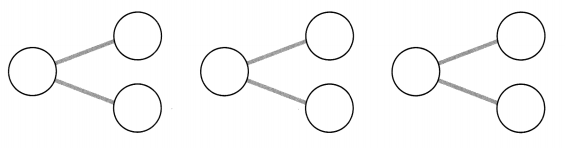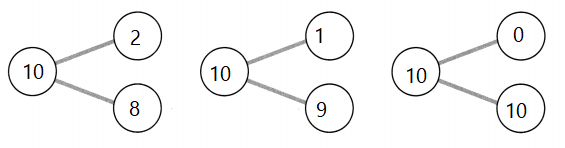# Math in Focus Grade 1 Chapter 2 Practice 1 Answer Key Making Number Bonds

This handy Math in Focus Grade 1 Workbook Answer Key Chapter 2 Practice 1 Making Number Bonds detailed solutions for the textbook questions.

## Math in Focus Grade 1 Chapter 2 Practice 1 Answer Key Making Number Bonds

Look at the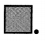Fill in the parts.

Example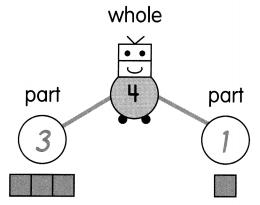Question 1.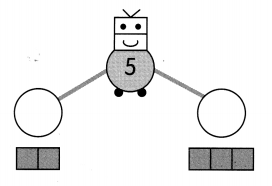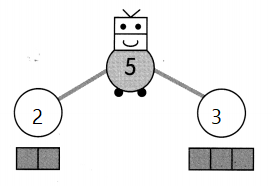Explanation:
2 + 3 = 5
The sum of 2 and 3 is 5
Adding left side blocks and right side blocks

Question 2.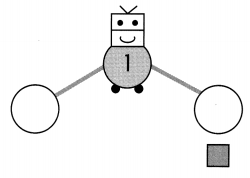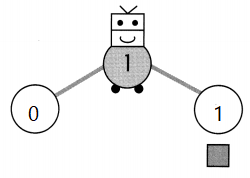Explanation:
0 + 1 = 1
The sum of 0 and 1 is 1
Adding left side blocks and right side blocks

Look at the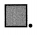Fill in the whole.

Example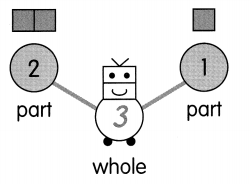Question 3.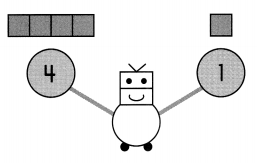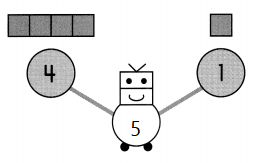Explanation:
4 + 1 = 5
The sum of 4 and 1 is 5
Adding left side blocks and right side blocks

Question 4.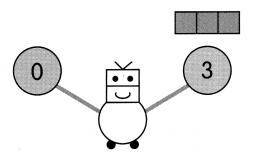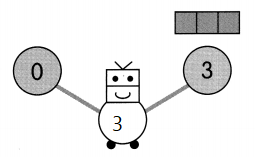Explanation:
0 + 3 = 3
The sum of 0 and 3 is 3
Adding left side blocks and right side blocks

Question 5.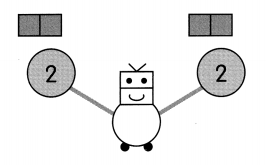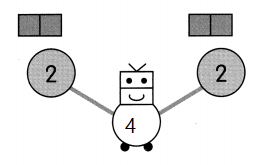Explanation:
2 + 2 = 4
The sum of 2 and 2 is 4
Adding left side blocks and right side blocks

Look at the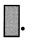.

Fill in the parts.

Example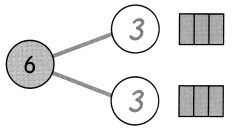Question 6.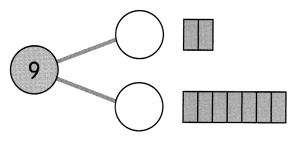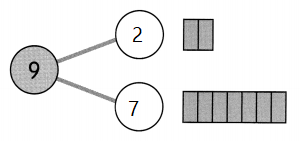Explanation:
The sum of 2 and 7 is 9
2 + 7 = 9
Adding top  blocks and the bottom blocks

Question 7.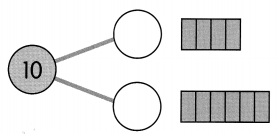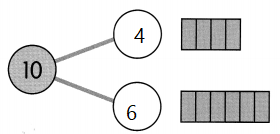Explanation:
6 + 4 = 10
6 and 4 are the counters of 10
the sum of 6 and 4 is 10
Adding top  blocks and the bottom blocks

Question 8.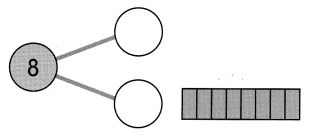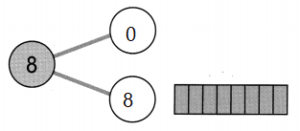Explanation:
8 + 0 = 8
Here the counters are 0 and 8
The sum of 0 and 8 is 8
Adding top  blocks and the bottom blocks

Question 9.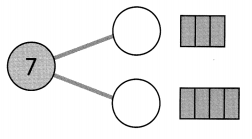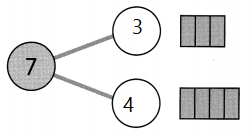Explanation:
3 + 4 = 7
The sum of 3 and 4 is 7
Here the counters are 3 and 4.
Adding top  blocks and the bottom blocks

Complete the number bonds. Fill in the blanks.

Question 11.
Are there any other numbers that make 10?

Example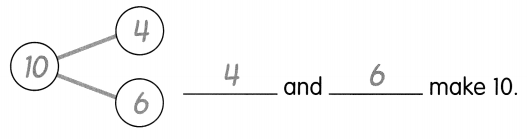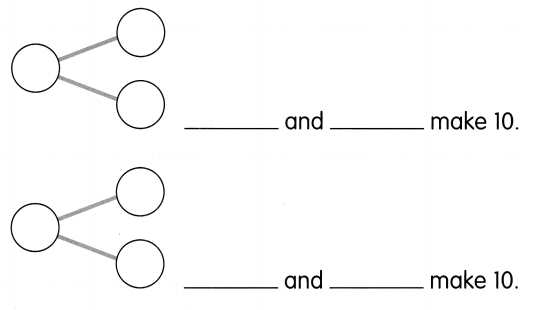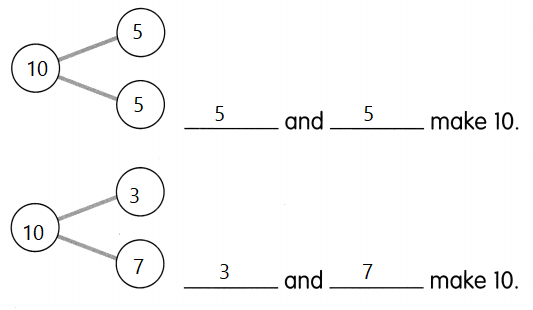Explanation:
5 + 5 = 10
7 + 3 = 10
The sum of 5 and 5 make 10
The sum of 3 and 7 make 10

Question 12.
Are there any other numbers that make 10?